# Nash theorem (in game theory)

(diff) ← Older revision | Latest revision (diff) | Newer revision → (diff)

A theorem on the existence of equilibrium points in a mixed extension of a finite non-cooperative game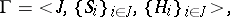where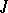and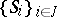are the finite sets of players and their strategies, respectively, and: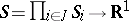is the pay-off function of player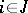(see also Games, theory of). It was established by J. Nash in . Let,, be the set of all probability measures on. Nash' theorem asserts that there is a measure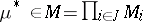for which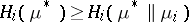for all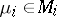,, wheredenotes the measure fromthat results from replacing the-th component of the vector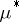by, and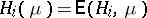. The known proofs of Nash' theorem rely on a fixed-point theorem.

How to Cite This Entry:
Nash theorem (in game theory). Encyclopedia of Mathematics. URL: http://encyclopediaofmath.org/index.php?title=Nash_theorem_(in_game_theory)&oldid=18406
This article was adapted from an original article by E.B. Yanovskaya (originator), which appeared in Encyclopedia of Mathematics - ISBN 1402006098. See original article